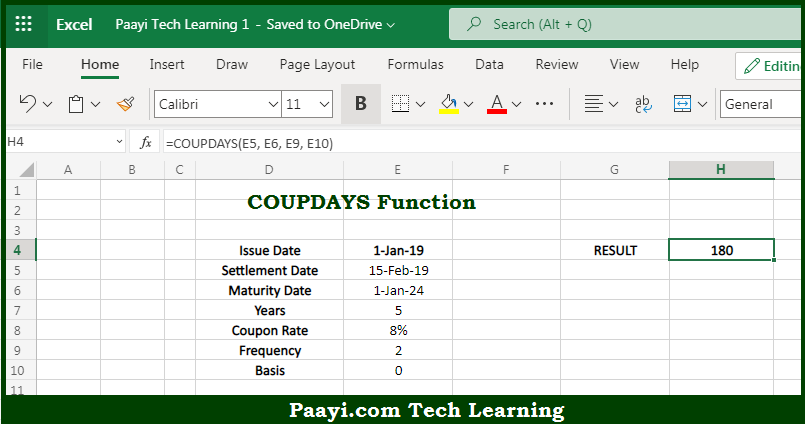# Learn How to Use Microsoft Excel COUPDAYS Function

Written by | 0 Comments | 489 Views

In this article, you will learn how to use the Microsoft Excel COUPDAYS function and its prime function in Microsoft Excel. You will also get to know the Microsoft Excel COUPDAYS function return value and syntax with the help of some examples.

Microsoft Excel COUPDAYS Function

The main purpose of the Microsoft Excel COUPDAYS function is to get the days from the coupon period inclusive of the settlement date. That implies, with the help of the COUPDAYS function you can able to return the number of days in the coupon period that includes the settlement date. So, with the help of the COUPDAYS function, you can able to get the days from the coupon period inclusive of the settlement date.

Return Value of COUPDAYS Function

The return value will be the calculated days.

Syntax of COUPDAYS Function

=COUPDAYS(settlement, maturity, frequency [basis])

Where the arguments:

• settlement: This is the settlement date of the security.
• maturity: This is the maturity date of the security.
• frequency: This is the number of coupon payments per year, where annual = 1, semi-annual = 2, and quarterly = 4.
• basis: This is the day count basis, default is 0 (optional).

## How to Use Microsoft Excel COUPDAYS Function?So we know that Microsoft Excel COUPDAYS function you can able to get the days from coupon period inclusive of the settlement date. That implies, with the help of the COUPDAYS function you can able to return the number of days in the coupon period that includes the settlement date. So, with the help of the COUPDAYS function, you can able to get the days from the coupon period inclusive of the settlement date.